# Community

Blog Events Webinars Tutorials Forum
×
Community Blog Understanding Negative Sampling in Graph Representation Learning

# Understanding Negative Sampling in Graph Representation Learning

6 researchers explain benefits and challenges of graph representation learning.

Download the "Cloud Knowledge Discovery on KDD Papers" whitepaper to explore 12 KDD papers and knowledge discovery from 12 Alibaba experts.

By Zhen Yang, Ming Ding, Chang Zhou, Hongxia Yang, Jingren Zhou, Jie Tang

In recent years, graph representation learning has been extensively studied. Graph representation learning can be used to generate continuous vector representations for all types of networks. However, it is still challenging to apply high-quality vector representations to large nodes effectively and efficiently. Most graph representation learning can be unified within a Sampled Noise Contrastive Estimation (SampledNCE) framework comprising of a trainable encoder to generate node embeddings, and a positive sampler and a negative sampler to sample positive and negative nodes respectively for any given node. The following figure shows the details. Prior arts usually focus on sampling positive node pairs, while the strategy for negative sampling is left insufficiently explored.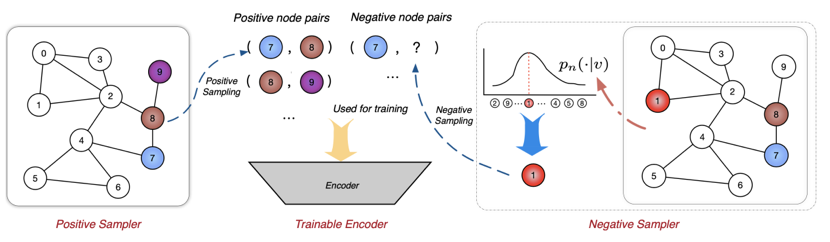To bridge the gap, we systematically analyze the role of negative sampling from the perspectives of both objective and variance, theoretically demonstrating that negative sampling is as important as positive sampling in determining the optimization objective and the resulted variance. First, we start from the following objective function for graph representation learning, and discuss a more generic graph representation learning form: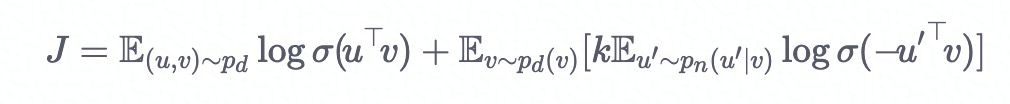where p_d denotes the estimated data distribution. p_n denotes negative sampling distribution. u→ and v→ denote the vector representations of nodes. σ(⋅) is the sigmoid function. We can calculate the optimal vector inner product in the ideal situation by using the following formula: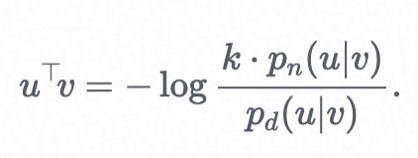The preceding formula shows that positive sampling distribution and negative sampling distribution have the same degree of impact on the optimization of the objective function.

We also analyze the effect of negative sampling in terms of variance. To minimize empirical errors, we sampled t positive sample pairs from positive sampling distribution and kT negative sample pairs from negative sampling distribution. Finally, we calculate the mean square error according to the theorem we proved: A random variable √T(θT−θ∗)T(θT−θ∗) gradually converges to a distribution that contains a zero-meaned vector and covariance matrix:

E[|(|θ_T-θ^* |)|^2 ]=1/T (1/(p_d (u│v) )-1+1/(kp_n (u│v) )-1/k)

According to the preceding formula, the mean square error is related to both the positive sampling distribution p_d and the negative sampling distribution p_n. We also defined the relationship between negative and positive sampling distributions, which is also the criterion for negative sampling. Accordingly, we propose that negative sampling distribution should have a positive but sublinear correlation with positive sampling distribution, that is, p_n (u│v)∝p_d (u│v)^α,0<α<1.

Under theoretical guidance, we propose an effective and scalable negative sampling strategy, namely, Markov chain Monte Carlo Negative Sampling (MCNS). This strategy uses self-contrastive approximation to estimate positive sampling distribution and uses Metropolis-Hastings to reduce the time complexity of negative sampling.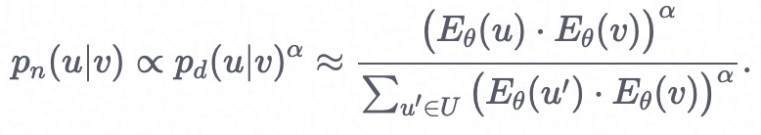Specifically, we estimate positive sampling distribution by using the inner product of the current encoder. This approach is similar to the technique in RotatE  and is very time-consuming. Each sampling operation requires O(n) time complexity, which makes it difficult to apply this approach to a large number of graphs. Our MCNS sampling approach uses the Metropolis-Hastings algorithm to solve this problem.

The following figure shows the MCNS framework that we propose. This framework traverses the graph through depth first traversal (DFS) to acquire the Markov chain of the last node. It then uses the Metropolis-Hastings algorithm to reduce the time complexity of the negative sampling process and imports the negative and positive samples to the encoder to acquire the vector representations of nodes. To train the model parameters, we replaced the binary cross-entropy loss function with hinge loss. The basic idea is to maximize the inner product of positive sample pairs and ensure that the inner product of negative sample pairs is smaller than the positive sample pairs by a certain threshold. The hinge loss is calculated as follows:

L = max(0,E_θ (v)⋅ E_θ (x)- E_θ (u)⋅ E_θ (v)+γ)

where (u,v) denotes positive sample pairs, (x,v) denotes negative sample pairs, and γ denotes a threshold, which is a hyperparameter.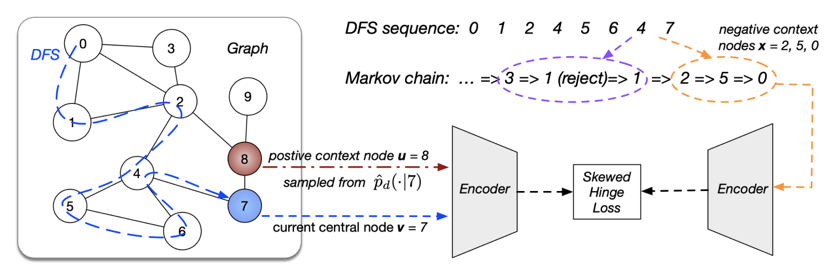In MCNS, we apply the Metropolis-Hastings algorithm to each node to reduce the time complexity of the negative sampling process. The q(y|x) distribution affects the convergence rate. q(y|x) is a 1:1 mixture of uniform sampling and sampling on K nearest neighboring nodes. The negative sampling process with MCNS is as follows: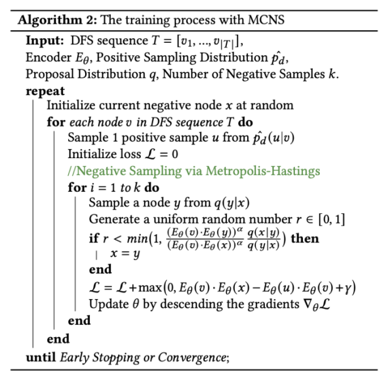To prove the effectiveness of MCNS, we conducted experiments on five datasets. In these experiments, we applied three typical graph representation learning algorithms to three commonly used graph representation learning tasks. These datasets covered 19 experimental setups, which included 8 negative sampling strategies collected from information retrieval , recommendation [3, 4], and knowledge graph embedding [5, 6]. The following table lists performance statistics collected from personalized recommendation tasks. In both situations where network embeddings and a Graph Neural Network (GNN) are used as encoders, MCNS always performs better than the other eight negative sampling strategies. Compared with the optimal baseline model, MCNS improves performance by 2% to 13%. In addition, we compared the efficiency of different negative sampling strategies on personalized recommendation tasks. As shown in the following figure, MCNS is more efficient than the other heuristic negative sampling strategies.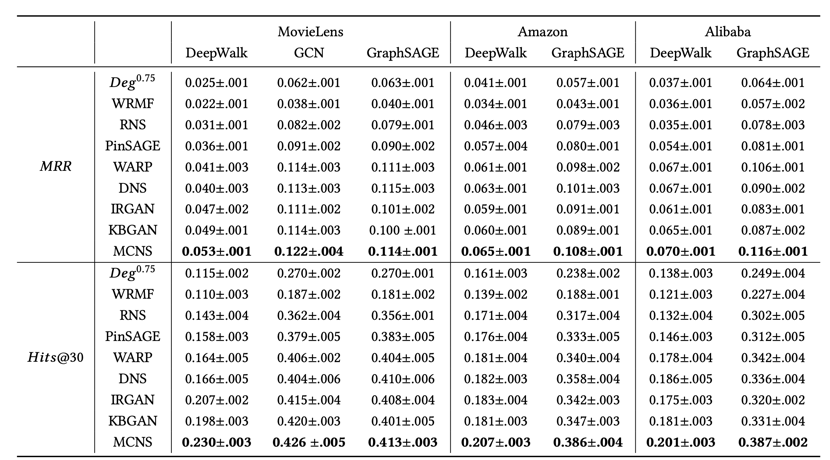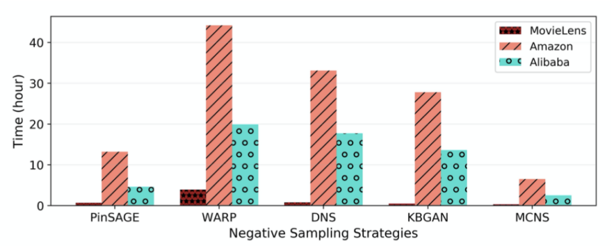Based on the Arxiv dataset, we evaluated the performance of different negative sampling strategies on link prediction tasks. The experiment results show that MCNS achieves varying degrees of performance improvement. In addition, based on the BlogCatalog dataset, we evaluated the performance of different models on node classification tasks. The experiment results show that MCNS surpasses all baselines in both situations where network embeddings and a GNN are used as encoders.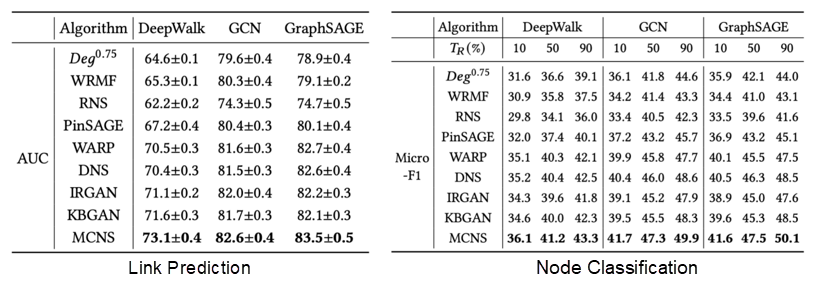Finally, we conducted additional experiments to gain deeper insights into MCNS. In the previous theoretical part, we analyzed negative sampling strategies in terms of objective function and variance. However, the following questions may arise: (1) Is it always helpful to sample more negative samples? (2) Why is our conclusion contradictory to the intuitive idea of "positive sampling on nearby nodes and negative sampling on distant nodes"?

To gain deeper insights into negative sampling, we designed two extended experiments to verify our theory. As shown in the left part of the following figure, if we collect more negative samples, the performance initially improves as the number of negative samples increases but then decreases. According to the mean square error calculation formula, in the beginning, more negative samples can reduce the variance, which in turn helps improve performance. However, after the optimal performance is reached, adding more negative samples degrades performance due to the occurrence of extra deviations. As shown in the right part of the following figure, negative sampling on distant nodes leads to disastrous consequences. We designed a strategy called inverseDNS. It selects the sample with the lowest score among candidate negative samples and uses it as the negative sample. In other words, it selects farther nodes for negative sampling. The experiment results show that MRR and Hits@30 decrease as the value of M is increased. This proves our theory.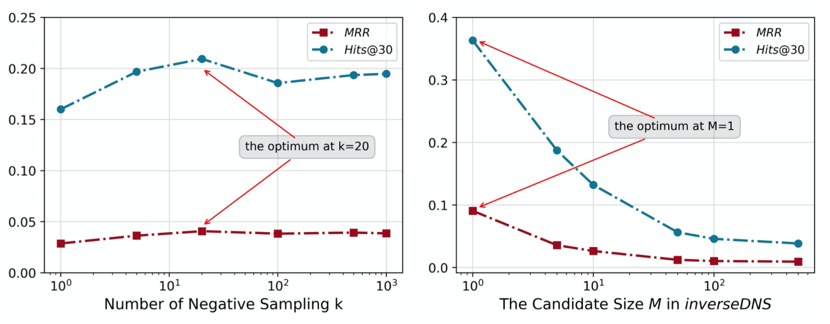## References

 Zhiqing Sun, Zhi-Hong Deng, Jian-Yun Nie, and Jian Tang. 2019. Rotate: Knowl- edge graph embedding by relational rotation in complex space. arXiv preprint arXiv:1902.10197 (2019).
 Jun Wang, Lantao Yu, Weinan Zhang, Yu Gong, Yinghui Xu, Benyou Wang, Peng Zhang, and Dell Zhang. 2017. Irgan: A minimax game for unifying generative and discriminative information retrieval models. In SIGIR'17. ACM, 515-524.
 Yifan Hu, Yehuda Koren, and Chris Volinsky. 2008. Collaborative filtering for implicit feedback datasets. In ICDM'08. IEEE, 263-272.
 Weinan Zhang, Tianqi Chen, Jun Wang, and Yong Yu. 2013. Optimizing Top-N Collaborative Filtering via Dynamic Negative Item Sampling. In SIGIR'13. ACM, 785-788.
 Liwei Cai and William Yang Wang. 2018. KBGAN: Adversarial Learning for Knowledge Graph Embeddings. In NAACL-HLT'18. 1470-1480.
 Yongqi Zhang, Quanming Yao, Yingxia Shao, and Lei Chen. 2019. NSCaching:Simple and Efficient Negative Sampling for Knowledge Graph Embedding. (2019), 614-625.

The views expressed herein are for reference only and don't necessarily represent the official views of Alibaba Cloud.

0 0 0
Share on

# Related Products

• ##Machine Learning Platform For AI

An end-to-end platform that provides various machine learning algorithms to meet your data mining and analysis requirements.

• ##Epidemic Prediction Solution

This technology can be used to predict the spread of COVID-19 and help decision makers evaluate the impact of various prevention and control measures on the development of the epidemic.

• ##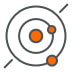Online Education Solution

This solution enables you to rapidly build cost-effective platforms to bring the best education to the world anytime and anywhere.

• ##Accelerated Global Networking Solution for Distance Learning

Alibaba Cloud offers an accelerated global networking solution that makes distance learning just the same as in-class teaching.